# Selina solutions for Concise Mathematics Class 7 ICSE chapter 17 - Symmetry (Including Reflection and Rotation) [Latest edition]

#### Chapters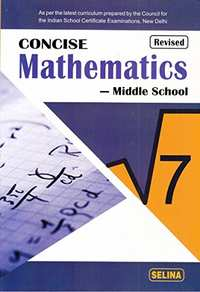## Chapter 17: Symmetry (Including Reflection and Rotation)

Exercise 17 (A)Exercise 17 (B)Exercise 17 (C)
Exercise 17 (A)

### Selina solutions for Concise Mathematics Class 7 ICSE Chapter 17 Symmetry (Including Reflection and Rotation) Exercise 17 (A)

Exercise 17 (A) | Q 1.1

In the figure given below, draw the line (s) of symmetry, if possible: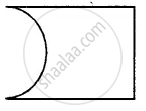Exercise 17 (A) | Q 1.2

In the figure given below, draw the line (s) of symmetry, if possible: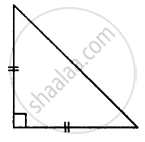Exercise 17 (A) | Q 1.3

In the figure given below, draw the line (s) of symmetry, if possible: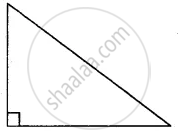Exercise 17 (A) | Q 1.4

In the figure given below, draw the line (s) of symmetry, if possible: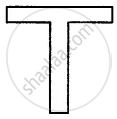Exercise 17 (A) | Q 1.5

In the figure given below, draw the line (s) of symmetry, if possible: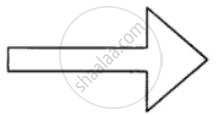Exercise 17 (A) | Q 1.6

In the figure given below, draw the line (s) of symmetry, if possible: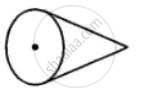Exercise 17 (A) | Q 1.7

In the figure given below, draw the line (s) of symmetry, if possible: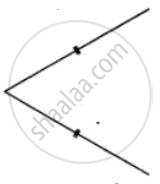Exercise 17 (A) | Q 1.8

In the figure given below, draw the line (s) of symmetry, if possible: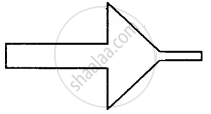Exercise 17 (A) | Q 2

Write capital letters A to Z of English alphabet; and in each case, if possible, draw the largest number of lines of symmetry.

Exercise 17 (A) | Q 3.1

By drawing a freehand sketch of the following, draw the line (s) of symmetry, if any:

a scalene triangle

Exercise 17 (A) | Q 3.2

By drawing a freehand sketch of the following, draw the line (s) of symmetry, if any:

an isosceles right-angled triangle

Exercise 17 (A) | Q 3.3

By drawing a freehand sketch of the following, draw the line (s) of symmetry, if any:

a rhombus

Exercise 17 (A) | Q 3.4

By drawing a freehand sketch of the following, draw the line (s) of symmetry, if any:

a kite-shaped figure triangle

Exercise 17 (A) | Q 3.5

By drawing a freehand sketch of the following, draw the line (s) of symmetry, if any:

a rectangle

Exercise 17 (A) | Q 3.6

By drawing a freehand sketch of the following, draw the line (s) of symmetry, if any:

a square

Exercise 17 (A) | Q 3.7

By drawing a freehand sketch of the following, draw the line (s) of symmetry, if any:

an isosceles

Exercise 17 (A) | Q 4.1

Draw a triangle with no line of symmetry. In case, if possible, represent the line/ lines of symmetry by dotted lines. Also, write the special name of the triangle drawn.

Exercise 17 (A) | Q 4.2

Draw a triangle with only one line of symmetry. In case, if possible, represent the line/ lines of symmetry by dotted lines. Also, write the special name of the triangle drawn.

Exercise 17 (A) | Q 4.3

Draw a triangle with exactly two lines of symmetry. In case, if possible, represent the line/ lines of symmetry by dotted lines. Also, write the special name of the triangle drawn.

Exercise 17 (A) | Q 4.4

Draw a triangle with exactly three lines of symmetry. In case, if possible, represent the line/ lines of symmetry by dotted lines. Also, write the special name of the triangle drawn.

Exercise 17 (A) | Q 4.5

Draw a triangle with more than three lines of symmetry. In case, if possible, represent the line/ lines of symmetry by dotted lines. Also, write the special name of the triangle drawn.

Exercise 17 (A) | Q 5.1

Draw a quadrilateral with no line of symmetry. In case, if possible, represent the line/ lines of symmetry by dotted lines. Also, write the special name of the quadrilateral drawn.

Exercise 17 (A) | Q 5.2

Draw a quadrilateral with only one line of symmetry. In case, if possible, represent the line/ lines of symmetry by dotted lines. Also, write the special name of the quadrilateral drawn.

Exercise 17 (A) | Q 5.3

Draw a quadrilateral with exactly two lines of symmetry. In case, if possible, represent the line/ lines of symmetry by dotted lines. Also, write the special name of the quadrilateral drawn.

Exercise 17 (A) | Q 5.4

Draw a quadrilateral with exactly three lines of symmetry. In case, if possible, represent the line/ lines of symmetry by dotted lines. Also, write the special name of the quadrilateral drawn.

Exercise 17 (A) | Q 5.5

Draw a quadrilateral with exactly four lines of symmetry. In case, if possible, represent the line/ lines of symmetry by dotted lines. Also, write the special name of the quadrilateral drawn.

Exercise 17 (A) | Q 5.6

Draw a quadrilateral with more than four lines of symmetry. In case, if possible, represent the line/ lines of symmetry by dotted lines. Also, write the special name of the quadrilateral drawn.

Exercise 17 (A) | Q 6

Construct an equilateral triangle with each side 6 cm. In the triangle drawn, draw all the possible lines of symmetry.

Exercise 17 (A) | Q 7

Construct a triangle ABC in which AB = AC = 5 cm and BC = 5.6 cm. If possible, draw its lines of symmetry.

Exercise 17 (A) | Q 8

Construct a triangle PQR such that PQ = QR = 5 .5 cm and angle PQR = 90°. If possible, draw its lines of symmetry.

Exercise 17 (A) | Q 9

If possible, draw a rough sketch of a quadrilateral which has exactly two lines of symmetry.

Exercise 17 (A) | Q 10

A quadrilateral ABCD is symmetric about its diagonal AC. Name three sides of this quadrilateral which are equal.

Exercise 17 (B)

### Selina solutions for Concise Mathematics Class 7 ICSE Chapter 17 Symmetry (Including Reflection and Rotation) Exercise 17 (B)

Exercise 17 (B) | Q 1.1

In the given figure, find the image of the point P in the line AB: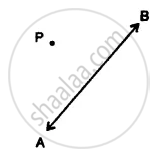Exercise 17 (B) | Q 1.2

In the given figure, find the image of the point P in the line AB: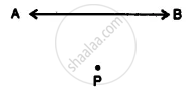Exercise 17 (B) | Q 2.1

In the given figure, find the image of the line segment AB in the line PQ: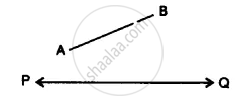Exercise 17 (B) | Q 2.2

In the given figure, find the image of the line segment AB in the line PQ: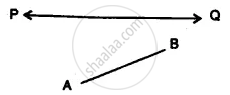Exercise 17 (B) | Q 3

Complete the following table:

 Point Reflection in x-axis y-axis origin (i) (8, 2) (ii) (5, 6) (iii) (4, −5) (iv) (6, −2) (v) (−3, 7) (vi) (−4, 5) (vii) (−2, −7) (viii) (−6, −3) (ix) (4, 0) (x) (−7, 0) (xi) (0, −6) (xii) (0, 7) (xiii) (0, 0)
Exercise 17 (B) | Q 4

A point P (7,3) is reflected in the x-axis to point P’. The point P’ is further reflected in v-axis to point P” Find :
(i) the coordinates of P’
(ii) the co-ordinates of P”
(iii) the image of P (7, 3) in origin.

Exercise 17 (B) | Q 5

A point A (- 5, 4) is reflected in y-axis to point B. The point B is further reflected in origin to point C. find :
(i) the co-ordinates of B
(ii) the co-ordinates of C
(iii) the image of A (- 5, 4) in x-axis.

Exercise 17 (B) | Q 6

The point P (3, – 8) is reflected in origin to point Q. The Point Q is further reflected in the x-axis to point R. Find :
(i) the co-ordinates of Q
(ii) the co-ordinates of R
(iii) the image of P (3, – 8) in the y-axis.

Exercise 17 (B) | Q 7

Each of the points A (3, 0), B (7, 0), C (- 8, 0), D (- 7, 0) and E (0, 0) is reflected in x-axis to points A’, B’, C’, D’ and E’ respectively. Write the co-ordinates of each of the image points A’, B’, C’, D’ and E’.

Exercise 17 (B) | Q 8

Each of the points A (0, 4), B (0, 10), C (0, – 4), D (0, – 6) and E (0, 0) is reflected in y-axis to points A’, B’, C’, D’ and E’ respectively. Write the co-ordinates of each of the image points A’, B’, C’, D’ and E’.

Exercise 17 (B) | Q 9

Each of the points A (0, 7), B (8, 0), C (0, − 5), D (− 7, 0) and E (0, 0) are reflected in origin to points A’, B’, C’, D’ and E’ respectively. Write the co-ordinates of each of the image points A’, B’, C’, D’ and E’.

Exercise 17 (B) | Q 10

Mark points A (4, 5) and B (− 5, 4) on a graph paper. Find A’, the image of A in x-axis and B’, the image of B in x-axis.
Mark A’ and B’ also on the same graph paper. Join AB and A’ B’ and find if AB = A’ B’?

Exercise 17 (B) | Q 11

Mark points A (6, 4) and B (4, – 6) on a graph paper.
Find A’, the image of A in y-axis and B’, the image of B in the y-axis. Mark A’ and B’ also on the same graph paper.

Exercise 17 (B) | Q 12

Mark points A (– 6, 5) and B (– 4, – 6) on a graph paper. Find A’, the image of A in origin and B’, the image of B in origin. Mark A’ and B’ also on the same graph paper. Join AB and A’ B’. Is AB = A’ B’?

Exercise 17 (C)

### Selina solutions for Concise Mathematics Class 7 ICSE Chapter 17 Symmetry (Including Reflection and Rotation) Exercise 17 (C)

Exercise 17 (C) | Q 1

How many lines of symmetry does a rhombus have?

Exercise 17 (C) | Q 2

What is the order of rotational symmetry of a rhombus?

Exercise 17 (C) | Q 3.1

Show that the following figure has two lines of symmetry and a rotational.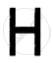Exercise 17 (C) | Q 3.2

Show that the following figure has two lines of symmetry and a rotational.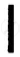Exercise 17 (C) | Q 3.3

Show that the following figure has two lines of symmetry and a rotational.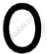Exercise 17 (C) | Q 3.4

Show that the following figure has two lines of symmetry and a rotational.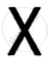Exercise 17 (C) | Q 4

Name a figure that has a line of symmetry but does not have any rotational symmetry.

Exercise 17 (C) | Q 5.1

In the following figure, draw all possible lines of symmetry and also write the order of rotational symmetry: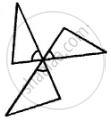Exercise 17 (C) | Q 5.2

In the following figure, draw all possible lines of symmetry and also write the order of rotational symmetry: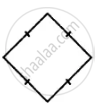Exercise 17 (C) | Q 5.3

In the following figure, draw all possible lines of symmetry and also write the order of rotational symmetry: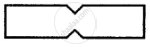Exercise 17 (C) | Q 5.4

In the following figure, draw all possible lines of symmetry and also write the order of rotational symmetry: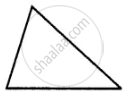Exercise 17 (C) | Q 5.5

In the following figure, draw all possible lines of symmetry and also write the order of rotational symmetry: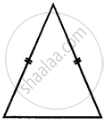## Chapter 17: Symmetry (Including Reflection and Rotation)

Exercise 17 (A)Exercise 17 (B)Exercise 17 (C)## Selina solutions for Concise Mathematics Class 7 ICSE chapter 17 - Symmetry (Including Reflection and Rotation)

Selina solutions for Concise Mathematics Class 7 ICSE chapter 17 (Symmetry (Including Reflection and Rotation)) include all questions with solution and detail explanation. This will clear students doubts about any question and improve application skills while preparing for board exams. The detailed, step-by-step solutions will help you understand the concepts better and clear your confusions, if any. Shaalaa.com has the CISCE Concise Mathematics Class 7 ICSE solutions in a manner that help students grasp basic concepts better and faster.

Further, we at Shaalaa.com provide such solutions so that students can prepare for written exams. Selina textbook solutions can be a core help for self-study and acts as a perfect self-help guidance for students.

Concepts covered in Concise Mathematics Class 7 ICSE chapter 17 Symmetry (Including Reflection and Rotation) are Concept for Idea of Rotational Symmetry, Observations of Rotational Symmetry of 2-d Objects. (900, 1200, 1800), Concept of Symmetry, Concept of Reflection Symmetry.

Using Selina Class 7 solutions Symmetry (Including Reflection and Rotation) exercise by students are an easy way to prepare for the exams, as they involve solutions arranged chapter-wise also page wise. The questions involved in Selina Solutions are important questions that can be asked in the final exam. Maximum students of CISCE Class 7 prefer Selina Textbook Solutions to score more in exam.

Get the free view of chapter 17 Symmetry (Including Reflection and Rotation) Class 7 extra questions for Concise Mathematics Class 7 ICSE and can use Shaalaa.com to keep it handy for your exam preparation